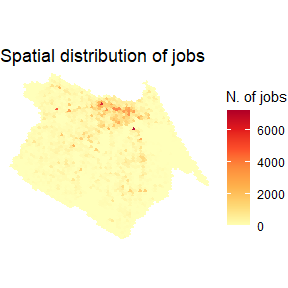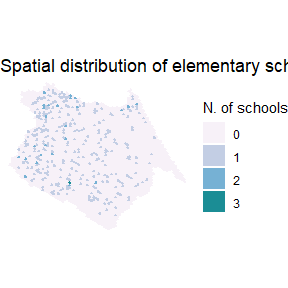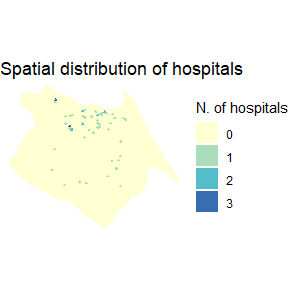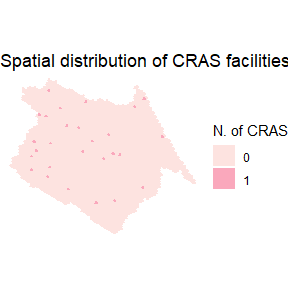# Mapping land use data

#### 2023-05-19

Spatial distribution of activities and urban services

# load libraries
library(aopdata)
library(data.table)
library(ggplot2)
library(sf)
library(scales)


year=2019,
geometry = T,
showProgress = F)

Map jobs Spatial distribution of jobs

ggplot() +
geom_sf(data=df, aes(fill=T001), color=NA, alpha=.9) +
scale_fill_distiller(palette = "YlOrRd", direction = 1) +
labs(title='Spatial distribution of jobs', fill="N. of jobs") +
theme_void()Map Schools Spatial distribution of elementary schools

ggplot() +
geom_sf(data=df, aes(fill=factor(E003)), color=NA, alpha=.9) +
scale_fill_brewer(palette = "PuBuGn", direction = 1) +
labs(title='Spatial distribution of elementary schools', fill="N. of schools") +
theme_void()Map Hospitals Spatial distribution of high-complexity health care facilities

ggplot() +
geom_sf(data=df, aes(fill=factor(S004)), color=NA, alpha=.9) +
scale_fill_brewer(palette = "YlGnBu", direction = 1)+
labs(title='Spatial distribution of hospitals', fill="N. of hospitals") +
theme_void()Map Centers for social assistance (CRAS) Spatial distribution of CRAS facilities

ggplot() +
geom_sf(data=df, aes(fill=factor(C001)), color=NA, alpha=.9) +
scale_fill_brewer(palette = "RdPu", direction = 1)+
labs(title='Spatial distribution of CRAS facilities', fill="N. of CRAS") +
theme_void()